### Practice Set 4.1 Financial Planning Class 10th Mathematics Part 1 MHB Solution

Practice Set 4.1
1. ‘Pawan Medical’ supplies medicines. On some medicines the rate of GST is 12%, then what…
2. On certain article if rate of CGST is 9% then what is the rate of SGST? and what is the…
3. ‘M/s. Real Paint’ sold 2 tins of lustre paint and taxable value of each tin is Rs.…
4. The taxable value of a wrist watch belt is Rs. 586. Rate of GST is 18%. Then what is…
5. The total value (with GST) of a remote-controlled toy car is Rs. 1770. Rate of GST is…
6. ‘Tiptop Electronics’ supplied an AC of 1.5 ton to a company. Cost of the AC supplied is…
7. Prasad purchased a washing-machine from 'Maharashtra Electronic Goods'. The discount of…
###### Practice Set 4.1
Question 1.

‘Pawan Medical’ supplies medicines. On some medicines the rate of GST is 12%, then what is the rate of CGST and SGST?

We know that CGST and SGST are components of GST.

CGST is always equal to SGST

i.e. GST = CGST + SGST

Let CGST and SGST be x.

Then GST = x + x

⇒ 12 = 2x

⇒ x = 6%

Hence CGST, SGST = 6%

Question 2.

On certain article if rate of CGST is 9% then what is the rate of SGST? and what is the rate of GST?

We know that CGST and SGST are components of GST.

CGST is always equal to SGST.

∴ CGST = SGST = 9%

We know that GST = CGST + SGST.

∴ GST = 9% + 9% = 18%

Question 3.

‘M/s. Real Paint’ sold 2 tins of lustre paint and taxable value of each tin is Rs. 2800. If the rate of GST is 28%, then find the amount of CGST and SGST charged in the tax invoice.

Given rate of GST = 28%

The taxable value of 1 tin = Rs.2800

∴ Taxable value of 2 tins = Rs.5600

We know that CGST and SGST are components of GST.

CGST is always equal to SGST

i.e. GST = CGST + SGST

Let CGST and SGST be x.

Then GST = x + x

⇒ 28 = 2x

⇒ x = 14%

Hence CGST, SGST = 14%

We know that CGST =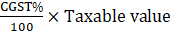⇒ CGST =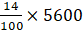= Rs.784

∵ CGST = SGST

∴ SGST = Rs.784

Question 4.

The taxable value of a wrist watch belt is Rs. 586. Rate of GST is 18%. Then what is price of the belt for the customer?

Given rate of GST = 18%

Taxable value of wrist watch belt = Rs.586

We know that CGST and SGST are components of GST.

CGST is always equal to SGST

i.e. GST = CGST + SGST

Let CGST and SGST be x.

Then GST = x + x

⇒ 18 = 2x

⇒ x = 9%

Hence CGST, SGST = 9%

We know that CGST =⇒ CGST =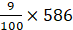= Rs.52.74

∵ CGST = SGST

∴ SGST = Rs.52.74

We know that price of a customer = Taxable value + CGST + SGST

∴ Price of belt for customer = 586 + 52.74 + 52.74

= 691.48

Question 5.

The total value (with GST) of a remote-controlled toy car is Rs. 1770. Rate of GST is 18% on toys. Find the taxable value, CGST and SGST for this toy-car.

We know that Total Value (with GST) = Taxable Value + GST

Given total value (with GST) = Rs. 1770

Let taxable value = x

∴ 1770 = x + 18% of x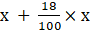= x + 0.18x

⇒ 1770 = 1.18x

⇒ x =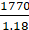= 1500

∴ x = Taxable Value = Rs. 1500

We know that CGST and SGST are components of GST.

CGST is always equal to SGST

i.e. GST = CGST + SGST

Let CGST and SGST be x.

Then GST = x + x

⇒ 18 = 2x

⇒ x = 9%

Hence CGST, SGST = 9%

We know that CGST =⇒ CGST =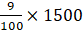= Rs. 135

∵ CGST = SGST

∴ SGST = Rs. 135

Question 6.

‘Tiptop Electronics’ supplied an AC of 1.5 ton to a company. Cost of the AC supplied is Rs. 51,200 (with GST). Rate of CGST on AC is 14%. Then find the following amounts as shown in the tax invoice of Tiptop Electronics.

(1) Rate of SGST

(2) Rate of GST on AC

(3) Taxable value of AC

(4) Total amount of GST

(5) Amount of CGST

(6) Amount of SGST

Given rate of CGST of AC = 14%

(1) We know that CGST and SGST are components of GST.

CGST is always equal to SGST.

∴ SGST = 14%

(2) We know that GST = CGST + SGST.

GST = 14% + 14% = 28%

(3) We know that Total Value (with GST) = Taxable Value + GST

Given total value (with GST) = Rs. 51, 200

Let taxable value = x

∴ 51200 = x + 28% of x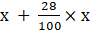= x + 0.28x

⇒ 51200 = 1.28x

⇒ x =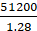= 40000

∴ x = Taxable Value = Rs. 40, 000

(4) We know that total GST = GST of Taxable value

∴ GST = 28% of 40, 000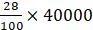= Rs. 11, 200

(5) We know that CGST and SGST are components of GST.

CGST is always equal to SGST

i.e. GST = CGST + SGST

Let CGST and SGST be x.

Then GST = x + x

⇒ 11200 = 2x

⇒ x = 5600

Hence CGST = Rs. 5600

(6) ∴ SGST = Rs. 5600

Question 7.

Prasad purchased a washing-machine from 'Maharashtra Electronic Goods'. The discount of 5% was given on the printed price of Rs. 40,000. Rate of GST charged was 28%. Find the purchase price of washing machine. Also find the amount of CGST and SGST shown in the tax invoice.

Discount = 5% of 40, 000 = Rs. 2000

∴ Taxable value of washing machine = 40,000 – 2000 = Rs. 38, 000

Given, rate of GST = 28%

We know that CGST and SGST are components of GST.

CGST is always equal to SGST

i.e. GST = CGST + SGST

Let CGST and SGST be x.

Then GST = x + x

⇒ 28 = 2x

⇒ x = 14%

∴ CGST, SGST = 14%

∴ CGST = 14% of 38, 000 = Rs. 5320

∴ SGST = Rs. 5320

∴ Purchase price of washing machine = 38000 + 5320 + 5320

= Rs. 48, 640

## PDF FILE TO YOUR EMAIL IMMEDIATELY PURCHASE NOTES & PAPER SOLUTION. @ Rs. 50/- each (GST extra)

SUBJECTS

HINDI ENTIRE PAPER SOLUTION

MARATHI PAPER SOLUTION
SSC MATHS I PAPER SOLUTION
SSC MATHS II PAPER SOLUTION
SSC SCIENCE I PAPER SOLUTION
SSC SCIENCE II PAPER SOLUTION
SSC ENGLISH PAPER SOLUTION
SSC & HSC ENGLISH WRITING SKILL
HSC ACCOUNTS NOTES
HSC OCM NOTES
HSC ECONOMICS NOTES
HSC SECRETARIAL PRACTICE NOTES

2019 Board Paper Solution

HSC ENGLISH SET A 2019 21st February, 2019

HSC ENGLISH SET B 2019 21st February, 2019

HSC ENGLISH SET C 2019 21st February, 2019

HSC ENGLISH SET D 2019 21st February, 2019

SECRETARIAL PRACTICE (S.P) 2019 25th February, 2019

HSC XII PHYSICS 2019 25th February, 2019

CHEMISTRY XII HSC SOLUTION 27th, February, 2019

OCM PAPER SOLUTION 2019 27th, February, 2019

HSC MATHS PAPER SOLUTION COMMERCE, 2nd March, 2019

HSC MATHS PAPER SOLUTION SCIENCE 2nd, March, 2019

SSC ENGLISH STD 10 5TH MARCH, 2019.

HSC XII ACCOUNTS 2019 6th March, 2019

HSC XII BIOLOGY 2019 6TH March, 2019

HSC XII ECONOMICS 9Th March 2019

SSC Maths I March 2019 Solution 10th Standard11th, March, 2019

SSC MATHS II MARCH 2019 SOLUTION 10TH STD.13th March, 2019

SSC SCIENCE I MARCH 2019 SOLUTION 10TH STD. 15th March, 2019.

SSC SCIENCE II MARCH 2019 SOLUTION 10TH STD. 18th March, 2019.

SSC SOCIAL SCIENCE I MARCH 2019 SOLUTION20th March, 2019

SSC SOCIAL SCIENCE II MARCH 2019 SOLUTION, 22nd March, 2019

XII CBSE - BOARD - MARCH - 2019 ENGLISH - QP + SOLUTIONS, 2nd March, 2019

HSC Maharashtra Board Papers 2020

(Std 12th English Medium)

HSC ECONOMICS MARCH 2020

HSC OCM MARCH 2020

HSC ACCOUNTS MARCH 2020

HSC S.P. MARCH 2020

HSC ENGLISH MARCH 2020

HSC HINDI MARCH 2020

HSC MARATHI MARCH 2020

HSC MATHS MARCH 2020

SSC Maharashtra Board Papers 2020

(Std 10th English Medium)

English MARCH 2020

HindI MARCH 2020

Hindi (Composite) MARCH 2020

Marathi MARCH 2020

Mathematics (Paper 1) MARCH 2020

Mathematics (Paper 2) MARCH 2020

Sanskrit MARCH 2020

Important-formula

THANKS